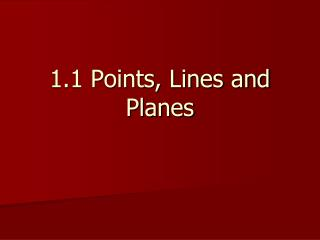DownloadDownload Presentation1.1 Points, Lines and Planes

# 1.1 Points, Lines and Planes

Télécharger la présentation## 1.1 Points, Lines and Planes

- - - - - - - - - - - - - - - - - - - - - - - - - - - E N D - - - - - - - - - - - - - - - - - - - - - - - - - - -
##### Presentation Transcript

1. 1.1 Points, Lines and Planes

2. Objectives: • Understand and use the basic undefined terms and defined terms of geometry. • Sketch the intersections of lines and planes.

3. Using Undefined terms and definition A definition uses known words to describe a new word. In geometry, some words such as point, line and plane are undefined terms or not formally defined.

4. A point has no dimension. It is usually represented by a small dot. Using Undefined terms and definition A Point A

5. A line exists in one dimension. It is usually represented by a straight line with two arrowheads to indicate that the line extends without end in two directions. In this book, lines are always straight lines. Using Undefined terms and definition l A B Line l or AB

6. A plane exists in two dimensions. It is usually represented by a shape that looks like a tabletop or wall. You must imagine that the plane extends without end even though the drawing of a plane appears to have edges. A M C B Using Undefined terms and definition Plane M or plane ABC

7. A few basic concepts . . . • Must be commonly understood without being defined. One such concept is the idea that a point lies on a line or a plane. • Collinear points are points that lie on the same line. • Coplanar points are points that lie on the same plane.

8. Name three points that are collinear Solution: D, E and F lie on the same line, so they are collinear. Ex. 1: Naming Collinear and Coplanar Points H G E F D

9. Name four points that are coplanar. Solution: D, E, F, and G lie on the same plane, so they are coplanar. Ex. 1: Naming Collinear and Coplanar Points H G E F D

10. Name three points that are not collinear. Solution: There are many correct answers. For instance, points H, E, and G do not lie on the same line; points G, D, and F. Ex. 1: Naming Collinear and Coplanar Points H G E F D

11. Another undefined concept in geometry is the idea that a point on a line is between two other points on the line. You can use this idea to define other important terms in geometry. Consider the line AB (symbolized by AB). More . . . l B A Line l or AB

12. The line segment or segment AB (symbolized by AB) consists of the endpoints A and B, and all points on AB that are between A and B. More . . . A B Segment AB l B A Line l or AB

13. The ray AB (symbolized by AB) consists of the initial point A and all points on AB that lie on the same side of A as point B. More . . . A B Ray AB l B A Line l or AB

14. Note that AB is the same as BA and AB is the same as BA. However, AB and BA are not the same. They have different initial points and extend in different directions. More . . . A B Ray BA l B A Line l or AB

15. If C is between A and B, then CA and CB are opposite rays. Like points, segments and rays are collinear if they lie on the same line. So, any two opposite rays are collinear. Segments, rays and lines are coplanar if they lie on the same plane. More . . . l B C A Line l or AB

16. K J L Ex. 2: Drawing lines, segments and rays • Draw three noncollinear points J, K, and L. Then draw JK, KL and LJ. Draw J, K and L Then draw JK

17. Ex. 2: Drawing lines, segments and rays • Draw three noncollinear points J, K, and L. Then draw JK, KL and LJ. K Draw KL J L

18. Ex. 2: Drawing lines, segments and rays • Draw three noncollinear points J, K, and L. Then draw JK, KL and LJ. K Draw LJ J L

19. Draw a line. Label 3 points on the line and name two pairs of opposite rays. Solution: Points M, N, and X are collinear and X is between M and N. So XM and XN are opposite rays. Ex. 3: Drawing Opposite Rays M X N

20. Draw another line through point X. Label points on the new line and name two pairs of opposite rays. Solution: Points P, Q, and X are collinear and X is between P and Q. So XP and XQ are opposite rays. Ex. 3: Drawing Opposite Rays M Q X P N

21. Goal 2: Sketching Intersections of Lines and Planes • Two or more geometric figures intersect if they have one or more points in common. The intersection of the figures is the set of points the figures have in common.

22. Sketch the figure described. A line that intersects a plane in one point Draw a plane and a line. Emphasize the point where they meet. Dashes indicate where the line is hidden by the plane Ex. 4: Sketching intersections

23. Sketch the figure described. Two planes that intersect in a line Draw two planes. Emphasize the line where they meet. Dashes indicate where one plane is hidden by the other plane. Ex. 4: Sketching intersections

24. Assignment: • Assignment: pp. 9-10 (#7-9, 13-18, 30-35, 38-46)